# Clockwise and Anti-Clockwise Turns

Clockwise and Anti-Clockwise Turns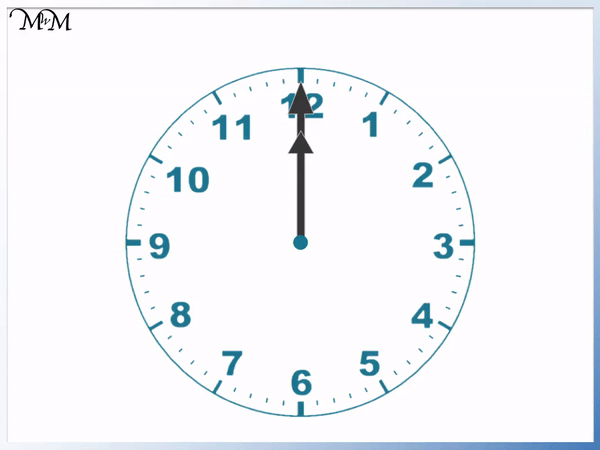• A clockwise turn is in the same direction that the hands of a clock turn.
• When the clock hands are facing upwards, a clockwise turn would begin by rotating to the right.
• An anti-clockwise turn is in the opposite direction to the movement of the hands on a clock.
• Anti-clockwise is also known as counterclockwise.

• When the clock hands are facing upwards, an anti-clockwise turn would begin by rotating to the left.
• A clock’s hands will never turn anti-clockwise, only clockwise.

A clockwise turn is in the same direction as the hands on a clock.

Anti-clockwise (or counterclockwise) is in the opposite direction to the hands on a clock.• This is a clockwise turn, which is a rotation in the same direction as the hands on a clock.
• Since the car begins by facing forwards, a clockwise turn begins with the front of the car turning to the right.
• A quarter turn means that the car rotates until it reaches the first quarter line shown.
• A quarter of the circle area has been shaded.
• The car was facing forwards and after a quarter turn clockwise, it is facing right.Supporting Lessons

#### Clockwise and Anti-Clockwise Turns: Accompanying Lesson Worksheet# What are Clockwise and Anti-Clockwise Turns?

The hands on a clock always rotate in the same direction as they move around the clock. This direction is called clockwise, or CW for short.

A clockwise turn is a turn in the same direction as the movement of the hands on a clock.If the front of an object is facing upwards (or forwards), then a clockwise turn starts by rotating to the right.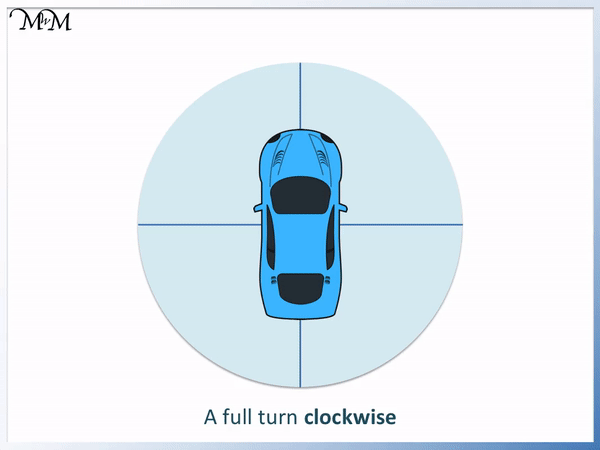The opposite direction to clockwise is called anti-clockwise (in English) or counterclockwise (in American English).

An anti-clockwise turn is a turn in the opposite direction to the movement of the hands on a clock.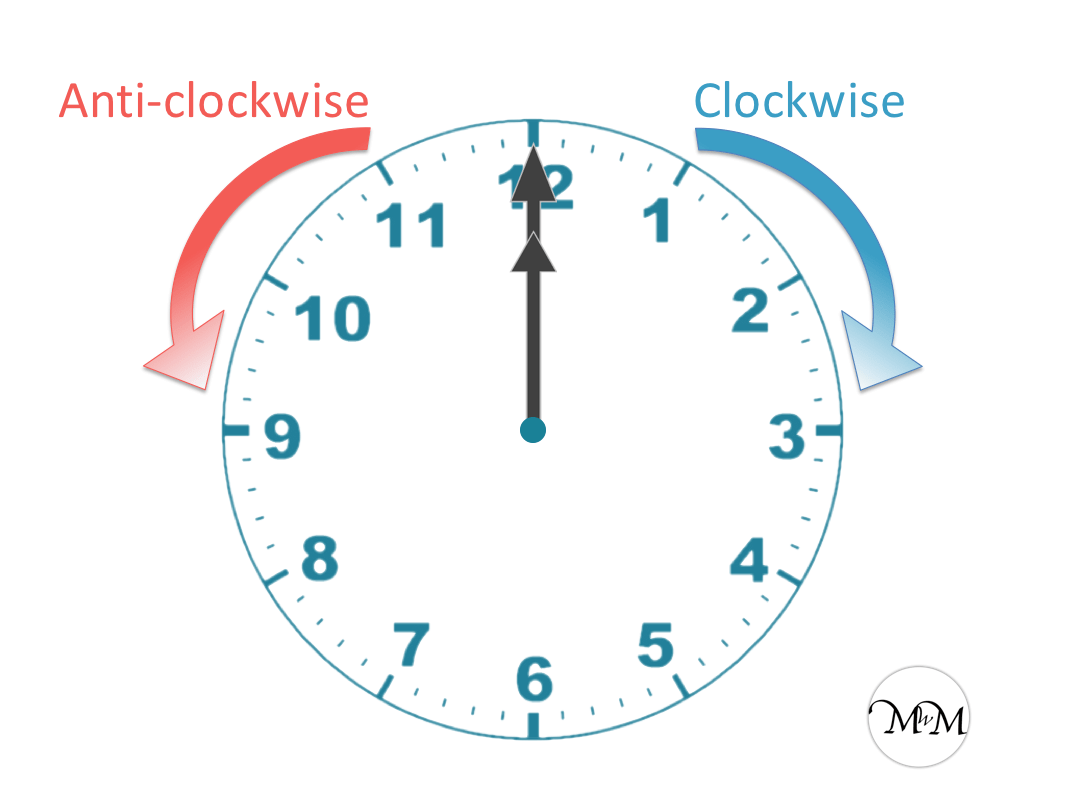If the front of an object is facing upwards (or forwards), then an anti-clockwise (or counterclockwise) turn starts by rotating to the left.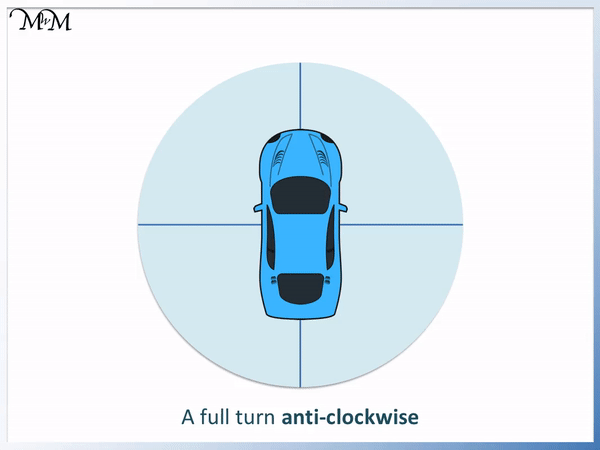## Quarter, Half, Three-Quarter and Full Turns in Clockwise and Anti-Clockwise Directions

Clockwise and anticlockwise turns are often taught in schools after students have mastered quarter, half, three-quarter and full turns.

Below is an animation of a car turning in the clockwise direction.

Each of the lines shown in the background divide the circle into quarters.The car is making a clockwise turn and so, the front of the car rotates in the same direction a the hands on a clock. The front of the car begins by rotating to the right.

As the front of the car faces right, it has made a quarter turn clockwise.As the front of the car faces downwards, it is in the opposite direction to the direction it started in. It has made half a turn clockwise.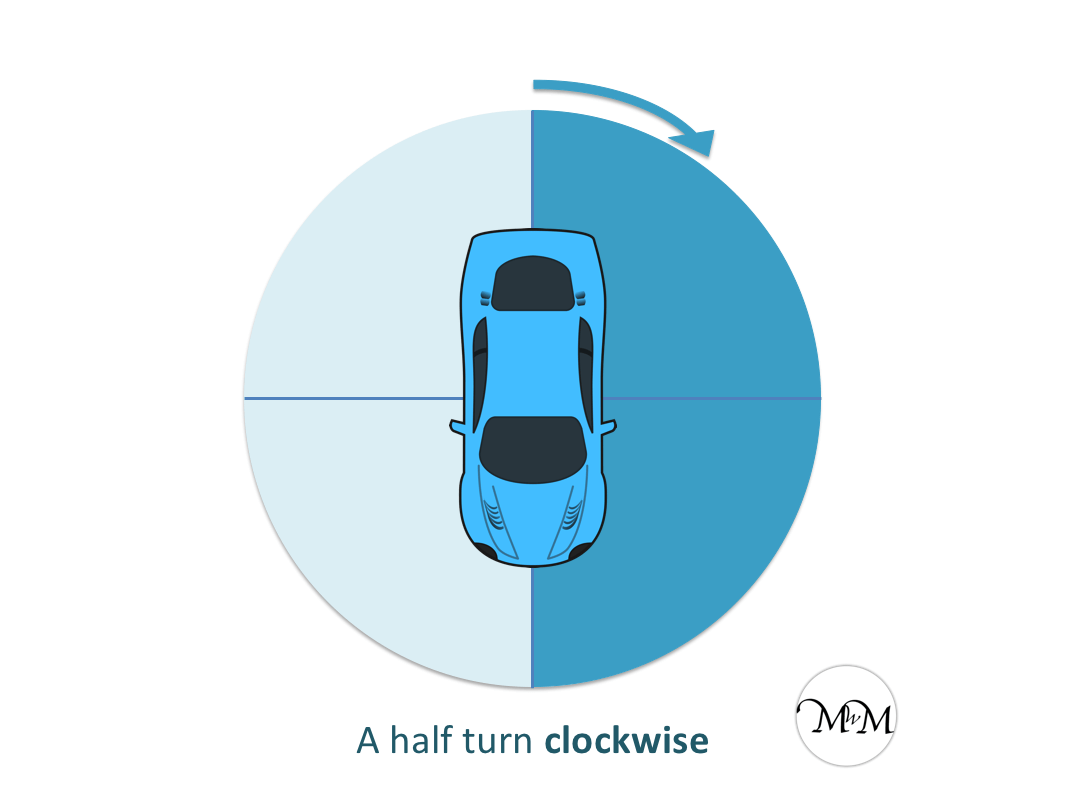As the car continues to rotate clockwise, it is facing left. It has made a three-quarter turn clockwise. The car is in line with the third of the quarter turn lines that it comes to.Once the car is facing back to where it started, we say it has made a full turn clockwise. We can see that the car has rotated through the whole circle area.

We will now compare quarter turns in both the clockwise and counterclockwise directions.

Here is a quarter turn clockwise.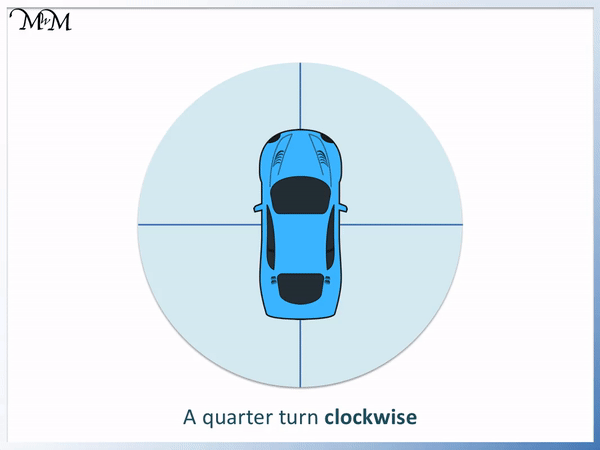The car finishes facing to the right because it started facing forwards.

Here is a quarter turn anti-clockwise. The car originally is facing forwards and so, the counterclockwise rotation begins by turning to the left.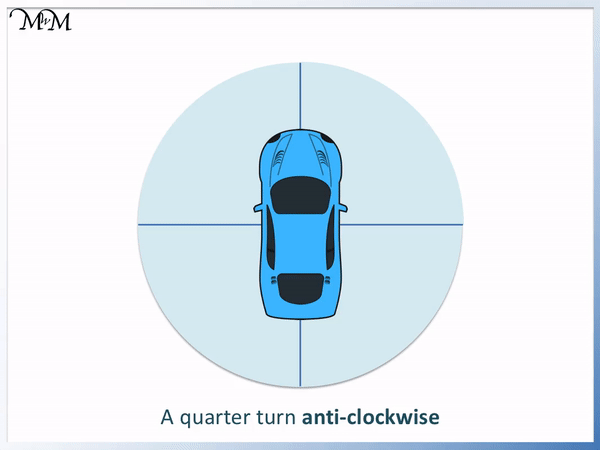The car finishes facing left.

We can see that a quarter turn clockwise leaves the car facing in the opposite direction to a quarter turn anticlockwise.

We will now look at a half turn clockwise.Here is a half turn anticlockwise.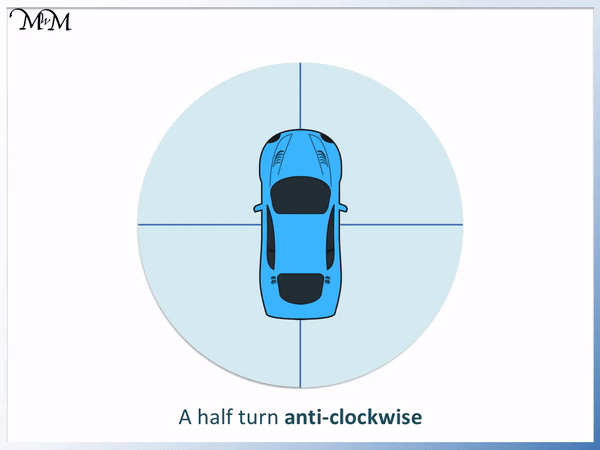We can see that both a half turn in either the counterclockwise or the clockwise direction result in the car facing in the opposite direction to which it started.

The final position of the car is the same in both half turns, however the direction taken to get there was different.

Below is an animation showing the car performing a half turn in both the clockwise and anticlockwise directions.Here is a three-quarter turn clockwise.The car rotates through three-quarters of a turn. We can see that three of the four quarters of the circle are shaded in.

The car is now facing left.

A three-quarter turn clockwise results in the car facing in the same final position as a one quarter turn counterclockwise.

Below is a three-quarter turn anticlockwise.We can see that a three-quarter turn anticlockwise results in the same final position as a one quarter turn clockwise.

Below is a full turn clockwise.

The car moves in the same direction as a clock until it has reached its starting point.The car was facing forwards to begin with and so, the car is facing forwards after a full turn.

Here is a car making a full turn anti-clockwise.We can see that a full turn counterclockwise results in the car facing in its original position.

A full turn clockwise results in the same final position as a full turn anti-clockwise. The direction traveled to get to this position was in the opposite direction.Now try our lesson on Perimeter of Irregular Shapes where we learn how to find the perimeter by adding the total outside length of shapes.error: Content is protected !!